# What Kind of Shape Is 1521 in?

### Today’s Puzzle:

1521 is a perfect square so if you had 1521 tiny squares, you could arrange them into a square perfectly. The following graphic has three sums on the right. The first sum shows that 1521 is the sum of the first 39 odd numbers. The last two sums prove that it is one more than eight times the 19th triangular number.Since 1521 is one more than eight times the 19th triangular number, it is also the 20th centered octagonal number. Today’s puzzle: How do we arrange 1521 tiny dots into a centered octagon?

I wanted to make a graphic of centered octagonal number 1521. I puzzled over how I could do that for several days. I decided to make it in Desmos. Since octagons are symmetrical, I knew that I could make 1/4 of it in Desmos and duplicate the other quarters in Paint. At first, I spent a lot of time typing ordered pairs in Desmos, until I realized how quickly I could make all the needed ordered pairs in Excel:

I made twenty 2-column ordered pairs in Excel. The top of each column was
(0, 0), (0, 1), (0, 2), etc . After I finished typing all of those in, I typed in the second ordered pair in each column from 2-20. I typed in the numerical value of (√2/2, (n-1) + √2/2) where n was the column number.

Then I used the drag function in Excel to complete columns 3 to 20. I dragged the first two ordered pairs in each column until I got an ordered pair where x = y. I made that last ordered pair be red.

Here is an example of how I build one column of ordered pairs:I found it easier to do a step for every column of ordered pairs before moving onto the next step. Since I wanted these points AND their inverses, I copied and pasted the non-red numbers from the x column into the y column and vice-versa.

Then I used the sort feature in Excel to get all the ordered pairs in ascending order of the x’s.

Lastly, I copied and pasted each column of ordered pairs in Desmos, and my quarter centered octagon quickly grew bigger and bigger with each paste. I found it quite enjoyable to do!

My octagon has lines and numbers on it and it wasn’t symmetrical so I needed to clean up the picture. I clicked on the wrench in Desmos, clicked on “Zoom Square” and removed all the checkmarks I saw. Then I clicked on the gear found above the ordered pairs in Desmos, clicked on the colored circles for each set of ordered pairs, and then clicked on the circle next to the word “lines,” and chose the solid line.

After I put the quarter octagon into Paint, I rotated copies of it every which way, added some words and brown lines until I got this beauty:It was so much fun to make, I encourage you to give it a try! I bet you can clearly see that 1521 is one more than eight times the 19th triangular number!

### Factors of 1521:

• 1521 is a composite number.
• Prime factorization: 1521 = 3 × 3 × 13 × 13, which can be written 1521 = 3²× 13².
• 1521 has at least one exponent greater than 1 in its prime factorization so √1521 can be simplified. Taking the factor pair from the factor pair table below with the largest square number factor, we get √1521 = (√9)(√169) = (3)(13) = 39.
• The exponents in the prime factorization are 1 and 1. Adding one to each exponent and multiplying we get (2 + 1)(2 + 1) = 3 × 3 = 9. Therefore 1521 has exactly 9 factors.
• The factors of 1521 are outlined with their factor pair partners in the graphic below.### More Facts about the Number 1521:

1521 is the sum of two squares:
36² + 15² = 1521.

1521 is the hypotenuse of two Pythagorean triples:
585-1404-1521, which is (5-12-13) times 117, and
1071-1080-1521, calculated from 36² – 15², 2(36)(15), 36² + 15².

From OEIS.org we learn that 1521 is sum of 4 distinct cubes in 3 different ways:
11³ +  5³ +  4³ +  1³ = 1521,
10³ +  8³ +  2³ +  1³ = 1521, and
9³ +  8³ +  6³ +  4³ = 1521.

We also learn that 1520 and 1521 are a Ruth-Aaron pair because they are consecutive numbers and the sum of their factors are equal to each other:
1520 = 2⁴·5·19 and 1521 = 3²·13², while
2+2+2+2+5+19 = 32=3+3+13+13.

# An Efficient Way to Quickly Find All the Odd Prime Numbers Less Than 1369

As I’ve used different sized grids to play with the Sieve of Eratosthenes, I’ve decided I like one size grid better than all the rest. It has six odd numbers across, but I repeat the first column on the right because of convenience. You already know the only even prime number is 2, so this grid can help you find all the rest of the primes up to 1369 = 37².Look at the grid. What are some things that you notice about it?

Square numbers are never prime, so why do I have them outlined on the grid? Why are some of them crossed out? Is there a pattern for that, too?

If you’ve done a sieve where you cross out all the multiples of the prime numbers in order, perhaps you’ve noticed that the first multiple to get crossed out that hasn’t been crossed out before is always the prime number squared.

Therefore, don’t start with the prime numbers. Start with their squares! The squares of each of the prime numbers and the next five odd multiples after those squares are listed in a box on the left of the paper. Put a dot in the corner of each of those multiples. Recognize the pattern they make and strike through those numbers with a colored pencil. A ruler will be helpful. Continue the same pattern down to the bottom of the grid. Then do the same thing with the next square of a prime number.  I’ve made a gif of these instructions being applied to a much smaller grid.make science GIFs like this at MakeaGif

It feels like I’m wrapping twinkling lights around a long sheet of cardboard!

Do try it on this much longer grid that goes to 1369. You’ll probably want to cut it out and glue or tape it together on the back. If this is an assignment, don’t cut off your name.

Read the following AFTER you’ve tried using the grid. I don’t want to spoil your sense of discovery!

To me, the lines drawn have a slope even if the lines are broken lines.
The slope for the 3s is undefined.
For the 5s, it’s +1; for the 7’s, it’s -1;
For the 11’s, it’s +2; for the 13’s, it’s -2;
For the 17’s, it’s +3; for the 19’s, it’s -3;
For the 23’s, it’s +4; for the 25’s, it’s -4; (You can skip the 25’s because they are already crossed out.)
For the 29’s, it’s +5; for the 31’s, it’s -5;
Cross out 37², and then you are done.

If the grid were longer, you could continue with the same pattern for as long as you want. I think it is pretty cool.

Now I’ll tell you a little bit about the number 1369:

• 1369 is a composite number and is a perfect square.
• Prime factorization: 1369 = 37 × 37 which can be written 1369 = 37²
• 1369 has at least one exponent greater than 1 in its prime factorization so √1369 can be simplified. Taking the factor pair from the factor pair table below with the largest square number factor, we get √1369 = (√37)(√37) = 37
• The exponent in the prime factorization is 2. Adding one to that exponent, we get (2 + 1) = 3. Therefore 1369 has exactly 3 factors.
• The factors of 1369 are outlined with their factor pair partners in the graphic below.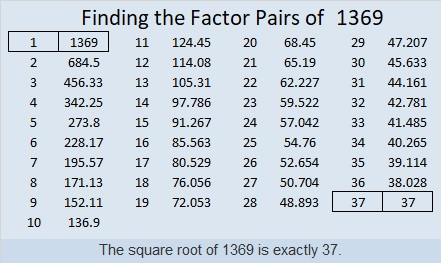1369 is the sum of two squares:
35² + 12² = 1369

1369 is the hypotenuse of two Pythagorean triples:
444-1295-1369 which is (12-35-37) times 37
840-1081-1369 calculated from 2(35)(12), 35² – 12², 35² + 12²

# What Kind of Shape is 1156 In?

One of the shapes that 1156 is in is a pentagon. Below you see 1156 tiny squares arranged into a pentagon.If you study the graphic above you will also see that 1156 is 1 more than five times the 21st triangular number.

The first few centered pentagonal numbers are 1, 6, 16, 31, 51, 76, 106. Double any centered pentagonal number 106 or greater, and you will get a number that can be represented as 552 in some base even if it’s a base nobody uses. Let’s see it at work here:
1156 × 2 = 2312, and 2312 is 552 in BASE 21

Why is that true? Because of the formula that calculates centered pentagonal numbers. For example, we know 1156 is the 22nd centered pentagonal number from
(5(21²) + 5(21) + 2(1))/2 = 1156

Written another way it looks like 1 more than 5 times the 21st triangular number:
5(21)(22)/2 + 1 = 1156

1156 is also a perfect square!

Not only is it a perfect square but it is the square of a composite number. The following two squares show how it can be made with multiple squares of its prime factors.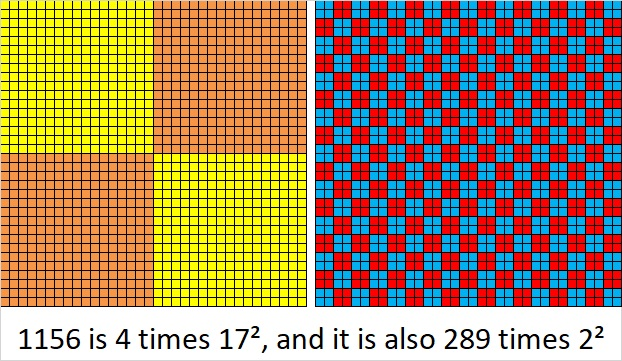• 1156 is a composite number.
• Prime factorization: 1156 = 2 × 2 × 17 × 17, which can be written 1156 = 2²× 17²
• The exponents in the prime factorization are 2 and 2. Adding one to each and multiplying we get (2 + 1)(2 + 1) = 3 × 3 = 9. Therefore 1156 has exactly 9 factors.
• Factors of 1156: 1, 2, 4, 17, 34, 68, 289, 578, 1156
• Factor pairs: 1156 = 1 × 1156, 2 × 578, 4 × 289, 17 × 68, or 34 × 34
• 1156 is a perfect square. √1156 = 34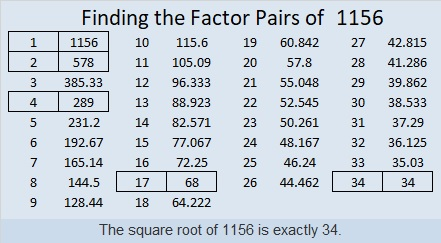Another shape where you can find 1156 are these two Pythagorean triple triangles:
644-960-1156 and 544-1020-1156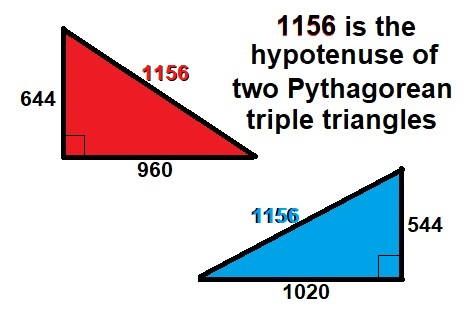1156 is also the sum of the twenty-four prime numbers from 5 to 101.

1156 is palindrome 1120211 in BASE 3 because 3⁶+3⁵+2(3⁴)+2(3²)+3+1=1156

Square number 1156 also looks like some other square numbers when it is written in these other bases:
It’s 961 in BASE 11 because 9(11²) + 6(11) + 1(1) = 1156,
484 in BASE 16 because 4(16²) + 8(16) + 4(1) = 1156,
400 in BASE 17 because 4(17²) = 1156,
169 in BASE 31 because 31² + 6(31) + 9(1) = 1156,
144 in BASE 32 because 32² + 4(32) + 4(1) = 1156,
121 in BASE 33 because 33² + 2(33) + 1(1) = 1156,
and 100 in BASE 34 because 34² = 1156

# 961 is a Perfect Square in More Ways Than One

961 is a perfect square. Its square root is 31. Look what happens when their digits are reversed. We still get a perfect square!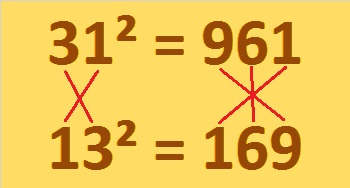The digits 1, 6, and 9 form six different 3-digit numbers when each of those digits is used only one time. Here are some square facts about each of those numbers:

169 = 13²
196 = 14²
619 = 310² – 309²
691 = 346² – 345²
916 = 30² + 4²
961 = 31²

Of course, 169 also equals 5² + 12²

So 961 is a perfect square forward, backward, and upside-down!

My friend, Muthu Yuvaraj, shared a couple of similar square facts with me: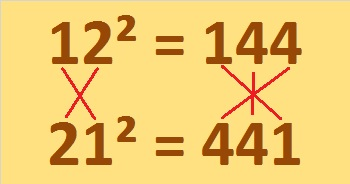andFascinating!

It is the sum of three consecutive square numbers:
313 + 317 + 331 = 961

It is the sum of five consecutive square numbers:
181 + 191 + 193 + 197 + 199 = 961

And it is the sum of the twenty-three prime numbers from 3 to 89.

It is palindrome 12321 in BASE 5 because 1(5⁴) + 2(5³) + 3(5²) + 2(5¹) + 1(5⁰) = 961

961 is a perfect square in base 10, and it looks like a perfect square in some other bases, too:
100 in BASE 31 because 1(31²) = 961
121 in BASE 30 because 1(30²) + 2(30¹) + 1(30⁰) = 961
144 in BASE 29 because 1(29²) + 4(29¹) + 4(29⁰) = 961
169 in BASE 28 because 1(28²) + 6(28¹) + 9(28⁰) = 961
441 in BASE 15 because 4(15²) + 4(15¹) + 1(15⁰) = 961
Remarkably, we saw 961, 169, 144, and 441 already in graphics for this post! So here’s one more graphic: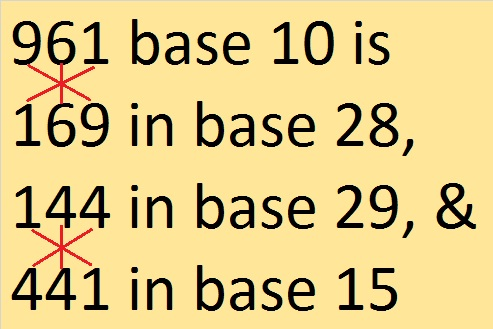• 961 is a composite number.
• Prime factorization: 961 = 31²
• The exponent in the prime factorization is 2. Adding one we get (2 + 1) = 3. Therefore 961 has exactly 3 factors.
• Factors of 961: 1, 31, 961
• Factor pairs: 961 = 1 × 961 or 31 × 31
• 961 is a perfect square. √961 = 31